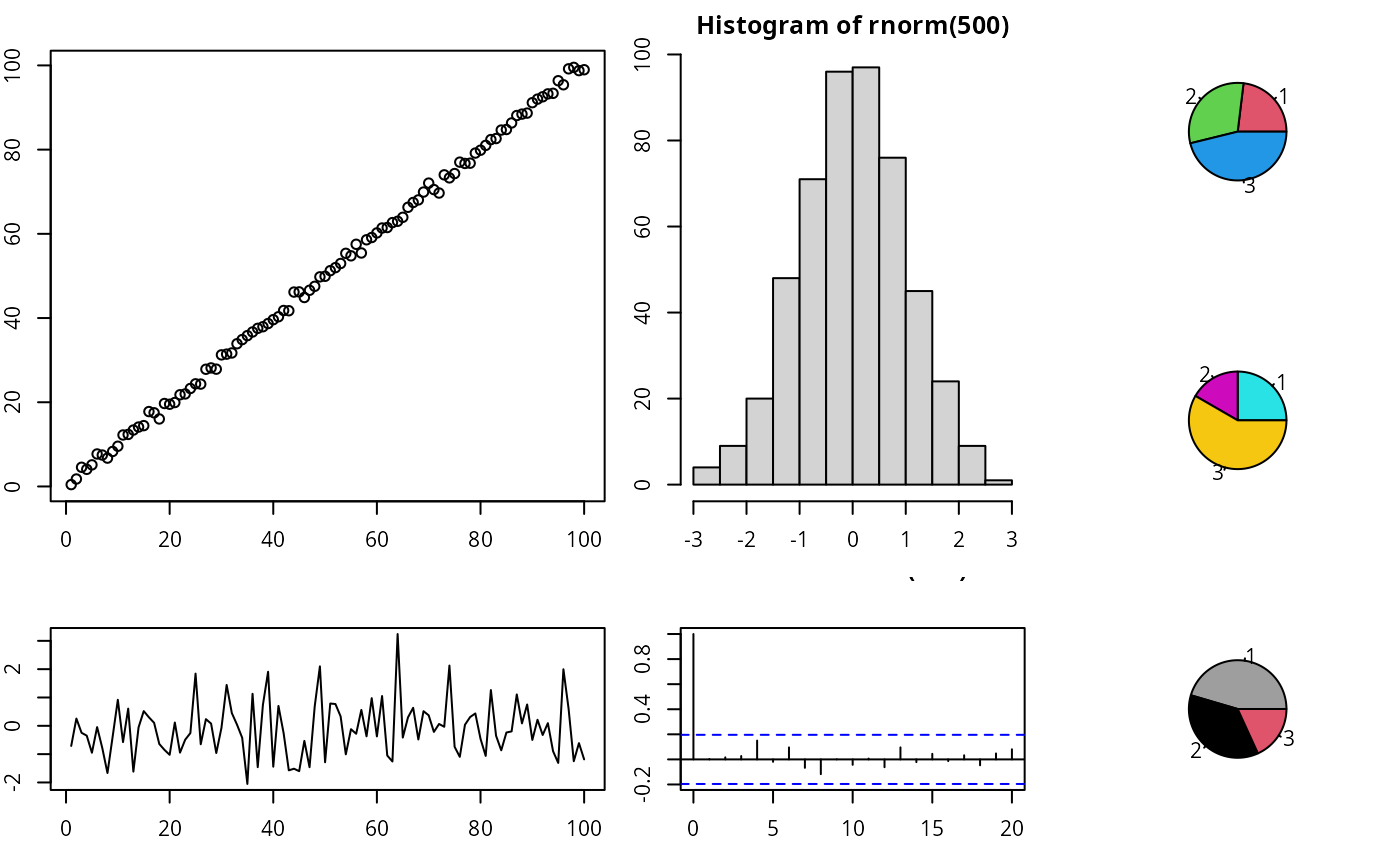Create custom layout.

lay_new(mat, widths = NULL, heights = NULL)

layCreate(mat, widths = NULL, heights = NULL)

## Arguments

mat a matrix specifying the location of the figures. See layout for more information. a vector of values for the relative heights of rows in mat. a vector of values for the relative heights of rows in mat.

## Examples

library(customLayout)
set.seed(123)
par(mar = c(3, 2, 2, 1))

# Prepare layout
lay  <- lay_new(matrix(1:4, nc = 2),
widths = c(3, 2),
heights = c(2, 1))
lay2 <- lay_new(matrix(1:3))
cl <- lay_bind_col(lay, lay2, widths = c(3, 1))
lay_set(cl) # initialize drawing area